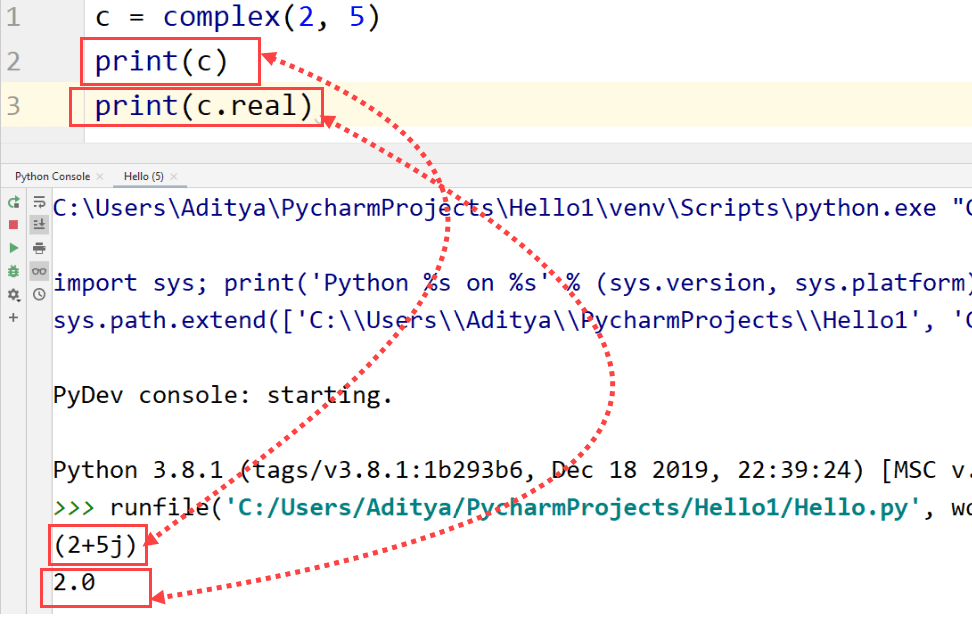# Python Numbers

## Python Numbers

Python has different data types for numbers. They are integers, floats and, complex Numbers. Those numbers are represented by “int”, “float” and “complex” respectively while declaring a variable.

Integers do not have a decimal point while floats have numbers after decimal, for example, 20 is an integer while 20.0 is a floating-point number. On the other hand, complex numbers are represented in the form “x +yj” where x and y are real and imaginary parts of the complex number.

In Python, as already discussed, type() is used to find the data type of a variable.

## ExampleA float is accurate up to 15 decimal points while an integer can be of any length. Integers are further divided into signed integers and long integers. Long integers are written normally like signed integers but are followed by an “L”. “L” can be either in lowercase or in uppercase.

Tips
Although, Python allows you to write a lowercase L while declaring long integers but you should use an uppercase L to avoid confusion.

## Conversion of Number Data types

In Python, numbers are converted into the different number data types internally. Sometimes, you might want to change the data type of the number according to the operator or parameters of the function.

In subtraction and addition, integers are coerced into floating point numbers implicitly if one of the numbers is a float.

## ExampleTo convert a float into integer, just use int(x) where x is a floating-point number. In this conversion, the float number gets converted into the smallest and nearest integer number.

## ExampleThe conversion of complex numbers into integers or floating-point decimals cannot be done like the integer to float conversion. This can be done in this way

## Example## Mathematical Functions

Python has pre-defined mathematical functions that can perform various operations on numbers.

• cmp(a, b)
This function is used to compare two numbers. If a < b, it returns –1; if a == b, it returns 0; if a > b, it returns 1.
• exp(a)
It calculates the exponential of ‘a’.
• ceil(a)
It finds the smallest integer which is not less than ‘a’. This function can be used on floating point decimals
• abs(a)
This function provides the absolute value of ‘a’, i.e., the positive distance between ‘a’ and zero.
• floor(a)
This function is used to find the largest integer that is not larger than ‘a’.
• sqrt(a)
This function finds the square root of ‘a’.
• log(a)
This will calculate the natural logarithmic value of ‘a’.
• log10(a)
This will find the base-10 algorithmic value of ‘a’.